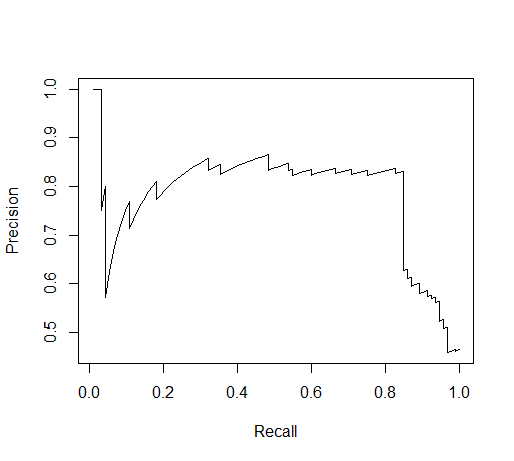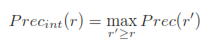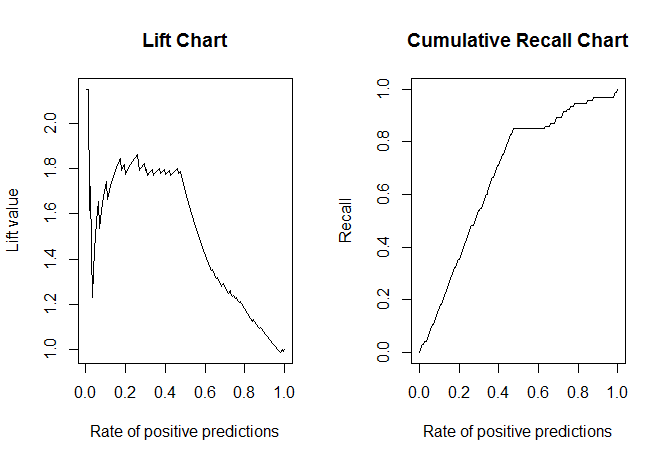## Saturday, March 28, 2015

### Predicting Fraudulent Transactions in R: Part 4. Model Criterion, Precision & Recall

Before we start creating classification models to predict fraudulent transactions, we need to understand how to evaluate model performance. Not only do we want to know if the particular transaction is predicted as fraudulent or not, we also want to know the confidence in that particular prediction (such as a probability of fraud).

To do this, we will use two measures, precision and recall. Beginning where we left off at the end of Part 3, start R and let us get started.

(This is a series from Luis Torgo's  Data Mining with R book.)

### Precision & Recall

Because we have a limited number of transactions we can inspect, k transactions out of 401146 total transactions, we can determine the precision and recall of the model on those k transactions. Those k top transactions contain the most likely fraud candidates predicted by the model.

Precision:
Within those k transactions, the proportion of frauds among them; also known as positive predictive value

Recall:
Among the fraudulent transactions, the proportion of frauds in the k transactions; also known as sensitivity

There are trade offs when making decisions concerning the level of recall and precision. It could be easy to use a large number of k transactions to capture all of the fraudulent transactions, but that would result in low precision as there will be a larger proportion of 'good' transactions in the k top transactions. Given the feasibility of inspecting k transactions, we want to maximize our resources in the transaction inspection activities. So when we inspect x transactions in t hours, and we manage to capture all the frauds in those x transactions, we will have accomplished our task. Even if a large proportion of those x transactions were valid transactions, we would value high recall in this situation.

### Performance Models

We now turn to evaluation of different inspection effort levels using different visual graphics. Some statistical models might be suited towards precision or towards recall. We would rank the class of interest (fraud) and interpolate the precision and recall values at different effort limits to determine those precision and recall values. Different effort limits will yield different precision and recall values, thus creating a visual performance model where we can see the optimal effort limits of high/low precision and recall values.

Using package "ROCR" that contains multiple functions for evaluating binary classifiers (yes/no, fraud/no fraud variables), including functions that calculate precision and recall, which we will then use to plot the performance model. Below, we load the "ROCR" library and the "ROCR.simple" data example with predictions in R. Next, in line 4, with the "prediction( )" function, we create "pred" using the "\$predictions" and the true values in "\$labels". Afterwards, we pass the "pred" prediction object to "performance( )" to obtain the precision and recall metrics. In line 5, we specify the "prec" and "rec" (precision and recall respectively) as arguments.

R Code:

 ```1 2 3 4 5 6 7``` ```> # precision and recall functions > library(ROCR) > data(ROCR.simple) > pred <- prediction(ROCR.simple\$predictions, ROCR.simple\$labels) > perf <- performance(pred, "prec", "rec") > plot(perf) > ```

Plotting the "perf" object in line 6 will plot the below 'sawtooth' graphic.Figure 1. Sawtooth Precision and Recall

As you can observe, at high levels of precision, the recall is not always high, and the same for high levels of recall. Note the axis limits of (0.5, 1.0) precision on the Y, and the (0.0, 1.0) range for recall on the X axis. This means that for 100% recall, where all the positives are captured, the precision (proportion of positives in the sample results) falls below 0.5,  due to capturing non-positives as well. Whereas for 100% precision, the recall falls to near zero, as not that many of the total positives are captured by the test. However, note the top right point, where the precision and recall both reach above 0.80- that is a good trade-off point if we were to take into account both precision and recall.

Evidently, the 'sawtooth' graph is not smooth at all. There is a method where we use the highest achieved precision level as we increase in recall value to smooth the curve. This interpolation of the precision takes the highest value of precision for a certain value of recall. As the value of recall increases, the maximum precision changes (decreases) to the maximum precision of precision values at greater recall values. This is described by the formula below, where r is the recall value, and r' are those values greater than r:Precision Interpolation

We show this smoothing by accessing the y values (precision) from the "performance( )" object.  Below is the R code for a function that plots the precision-recall curve with interpolation of the precision values. Line 3 checks if the "ROCR" package is loaded, and lines 4 and 5 are familiar, as they create the "prediction( )" and "performance( )" objects.

R Code:
 ``` 1 2 3 4 5 6 7 8 9 10 11 12 13 14``` ```> # precision smoothing > PRcurve <- function(preds, trues, ...) { + require(ROCR, quietly=T) + pd <- prediction(preds, trues) + pf <- performance(pd, "prec","rec") + pf@y.values <- lapply(pf@y.values, function(x) rev(cummax(rev(x)))) + plot(pf, ...) + } > > # plot both graphics side by side > par(mfrow=c(1,2)) > plot(perf) > PRcurve(ROCR.simple\$predictions, ROCR.simple\$labels) > ```

Line 6 accesses the "pf@y.values" and takes the cumulative maximum value of the reverse ordered y values, reverses them back, and replaces the original y values. The "cummax( )" function returns a vector with the maximum value in order by index (first, second, third value). By reversing the y values, we start decreasing at recall 1.0, and take the maximum value until we get another higher precision value, and reverse the order afterwards. Starting from line 11, we plot the two curves side by side (1 row, 2 columns) by calling the previous 'sawtooth' "perf" object and the "PRcurve" function.Figure 2. 'Sawtooth' and Interpolated Precision Precision-Recall Curves

Note how the smooth curve has all the highest possible precision values at increasing recall values. Also, in line 6, we use "lapply( )" to apply the function over the y values so we can pass multiple sets of y values with this "PRcurve" function.

### Fraudulent Transactions

So how does this apply to inspecting frauds? We know from the beginning that we aim to increase our recall metric to capture as many total frauds as possible for optimal efficiency. Last post we talked about transaction outliers and their outlier score. With the outlier scores, we can set a limit by establishing a threshold for the outlier score where a transaction is predicted as a fraud or non-fraudulent. That way, we have the predicted values. Additionally, we can compare the inspected results when we run the inspected values through the model and compare them with the predicted fraud status and the inspected status for precision and recall values.

### Lift Charts

Lift charts provide more emphasis on recall, and will be more applicable to evaluating fraudulent model transactions. These charts are different as they include the rate of positive predictions (RPP) on the X axis and the Y axis displays the recall value divided by the RPP. The RPP is the number of positive class predictions divided by the total number of test cases. For example, if there are 5 frauds predicted (though not all might be true frauds) out of 20 test cases, then the RPP is 0.25.

Again, the "ROCR" package includes the necessary functions to obtain the lift chart metrics.   We still use the "\$predictions" and "\$labels", but now use "lift" and "rpp" as our Y and X axes in the "performance( )" function in line 3. For the cumulative recall function, "CRchart", we use the same format as the "PRcurve" function but similarly substitute in "rec" for recall and "rpp" for rate of positive predictions.

R Code:
 ``` 1 2 3 4 5 6 7 8 9 10 11 12 13 14 15 16``` ```> # lift chart > pred <- prediction(ROCR.simple\$predictions, ROCR.simple\$labels) > perf <- performance(pred, "lift", "rpp") > par(mfrow=c(1,2)) > plot(perf, main="Lift Chart") > > # cumulative chart with rate of positive predictions > CRchart <- function( preds, trues, ...) { + require(ROCR, quietly=T) + pd <- prediction(preds, trues) + pf <- performance(pd, "rec", "rpp") + plot(pf, ...) + } > CRchart(ROCR.simple\$predictions, ROCR.simple\$labels, + main='Cumulative Recall Chart') > ```

This yields two graphs side by side:Figure 3. Lift Chart and Cumulative Recall Chart

The more close the cumulative recall curve is to the top left corner of the graph, the better the indication from the model. While lift charts contain the comparison of recall and RPP, the cumulative recall and RPP curve applies more here. It shows the recall value with changing inspection effort, where the number of cases are tested and determined to be frauds. At 1.0, all cases were tested, and therefore, all frauds were captured, showing 1.0 recall.

Next we will be exploring normalized distance to obtain the outlier score with which we will determine the threshold and evaluate the many models' recall metrics. Stay tuned, folks!